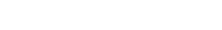# Mathew Gluck | Mathematics | SIU

HomeCONTACT

SIU.EDU

## Mathew Gluck

### Assistant ProfessorP: 618.453.6593
E: mathew.gluck@siu.edu
O: Neckers 391

Assistant Professor; Ph.D. Mathematics, University of Florida

### Research Interests

1. Nonlinear elliptic partial differential and integral equations arising in geometry, biology and physics
2. Extremal problems related to sharp Sobolev and Hardy-Littlewood-Sobolev inequalities
3. Calculus of variations
4. Applied and computational mathematics and data science

### Publications

1. Infinitely many solutions to a conformally invariant integral equation on Rn (2022) Nonlinear Analysis, 214.
2. (with A. Kolesnikov) Applied Mathematics Laboratory: a course-based research internship (2022) The Mathematics Enthusiast, 19 no.3, pp. 785{793.
3. Experimental Mathematics: a course-based research experience in machine learning (2022) The Mathematics Enthusiast, 19 no.3, pp. 822{832.
4. Classification of solutions to a system of nth order equations on Rn (2020) Communications in Pure & Applied Analysis 19 (12) pp. 5413{5436.
5. Subcritical approach to conformally invariant extension operators on the upper half space. J. Funct. Anal. 278 (2020) no. 1.
6. (with Meijun Zhu) An extension operator on bounded domains and applications (2019) Cal. of Var PDE. 58 (2) pp. 79{106.
7. (with Lei Zhang) Classification of solutions to a system of critically nonlinear elliptic equations on Euclidean half-space, J. Part. Diff. Eq. 28 No. 1 (2015), pp. 74{94.
8. (with Ying Guo and Lei Zhang), A Harnack-type inequality for a prescribed-curvature equation on a domain with boundary, Advanced Nonlinear Studies, 14 (2014) , pp. 709{746.
9. Asymptotic behavior of blow up solutions to a class of prescribing Gauss curvature equations, Nonlinear Analysis 75 (2012), pp. 5787{5796.